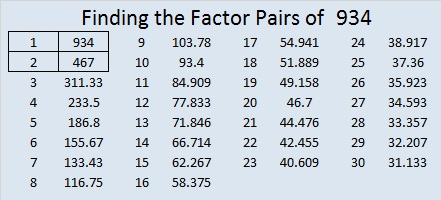# 934 and Level 2

I hope you are having a happy Halloween at your house. This puzzle is only a level 2. I’m confident that you can find where all the factors from 1 to 10 go in both the first column and the top row.Print the puzzles or type the solution on this excel file: 10-factors-932-941

Now let me tell you a little about the number 934:

934 is palindrome 424 in BASE 15 because
4(15²) + 2(15¹) + 4(15º) = 934

• 934 is a composite number.
• Prime factorization: 934 = 2 × 467
• The exponents in the prime factorization are 1 and 1. Adding one to each and multiplying we get (1 + 1)(1 + 1) = 2 × 2 = 4. Therefore 934 has exactly 4 factors.
• Factors of 934: 1, 2, 467, 934
• Factor pairs: 934 = 1 × 934 or 2 × 467
• 934 has no square factors that allow its square root to be simplified. √934 ≈ 30.56141Here are some mathematical posts I saw on Twitter to celebrate Halloween:

Halloween wishes to all mathematical types out there 😱 pic.twitter.com/0Jyk9IBNmp